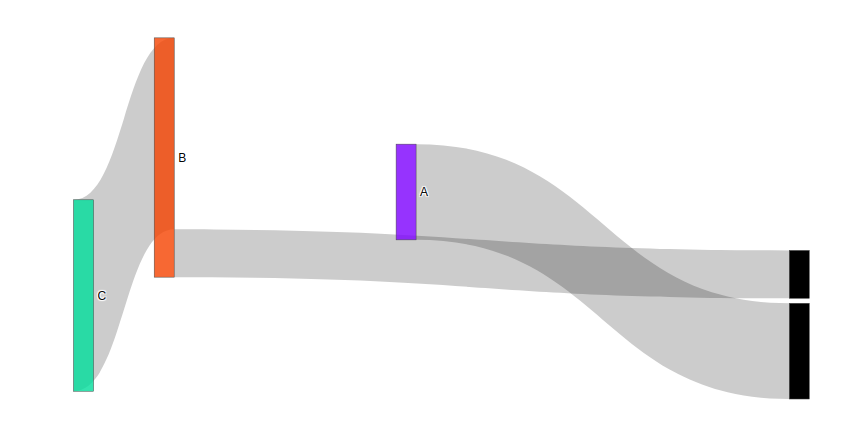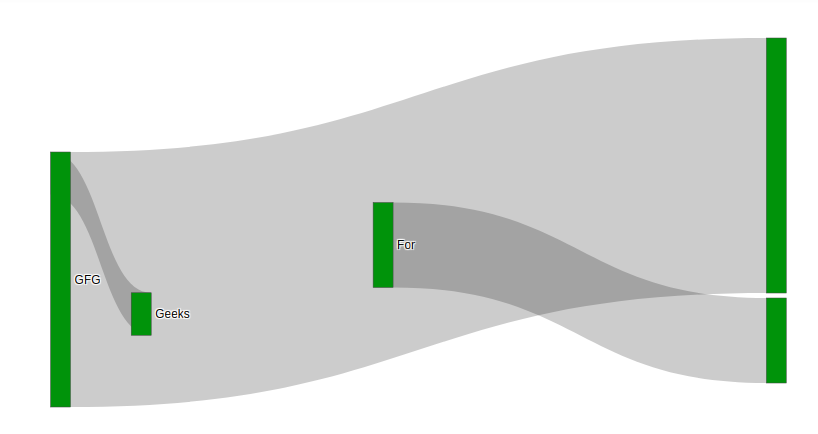# Define Node position in Sankey Diagram in plotly

Plotly is a Python library which is used to design graphs, especially interactive graphs. It can plot various graphs and charts like histogram, barplot, boxplot, spreadplot and many more. It is mainly used in data analysis as well as financial analysis. plotly is an interactive visualization library.

Sankey Diagram is used to visualize the flow by defining the source node and target node. Value parameters is used to set the flow volume. There are basically arrangement for defining a node position. There are –

• perpendicular
• freeform
• fixed

The node position can be set by setting the node.x and node.y value. let’s see the below example for better understanding.

Example 1:

## Python3

 `import` `plotly.graph_objects as go ` ` `  ` `  `plot ``=` `go.Figure(go.Sankey( ` `    ``node ``=` `{ ` `        ``"label"``: [``"A"``, ``"B"``, ``"C"``], ` `        ``"x"``: [``0.5``, ``0.2``, ``0.1``], ` `        ``"y"``: [``0.4``, ``0.3``, ``0.7``], ` `        ``'pad'``:``5``}, ` `    ``link ``=` `{ ` `        ``"source"``: [``1``, ``0``, ``1``], ` `        ``"target"``: [``2``, ``3``, ``4``], ` `        ``"value"``: [``4``, ``2``, ``1``]})) ` ` `  `plot.show()`

Output:Example 2:

## Python3

 `import` `plotly.graph_objects as go ` ` `  ` `  `plot ``=` `go.Figure(go.Sankey( ` `    ``node ``=` `{ ` `        ``"label"``: [``"Geeks"``, ``"For"``, ``"Geeks"``, ``"GFG"``], ` `        ``"x"``: [``0.5``, ``0.2``, ``0.1``, ``0.9``], ` `        ``"y"``: [``0.6``, ``0.8``, ``0.7``], ` `        ``"color"``: ``"green"``, ` `        ``'pad'``:``5``}, ` `    ``link ``=` `{ ` `        ``"source"``: [``3``, ``2``, ``1``], ` `        ``"target"``: [``5``, ``3``, ``7``], ` `        ``"value"``: [``6``, ``1``, ``2``]})) ` ` `  `plot.show()`

Output:Whether you're preparing for your first job interview or aiming to upskill in this ever-evolving tech landscape, GeeksforGeeks Courses are your key to success. We provide top-quality content at affordable prices, all geared towards accelerating your growth in a time-bound manner. Join the millions we've already empowered, and we're here to do the same for you. Don't miss out - check it out now!

Previous
Next